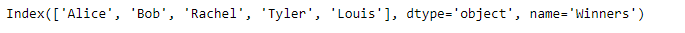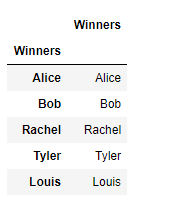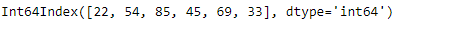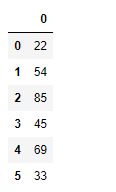Skip to content
Related Articles
Python | Pandas Index.to_frame()
• Last Updated : 24 Dec, 2018

Python is a great language for doing data analysis, primarily because of the fantastic ecosystem of data-centric python packages. Pandas is one of those packages and makes importing and analyzing data much easier.

Pandas` Index.to_frame()` function create a dataFrame from the given index with a column containing the Index. By default, the original Index is reused in the new dataframe. To reinforce a new index for the newly created dataframe, we set the index parameter of the function to be false.

Syntax: Index.to_frame(index=True)

Parameters :
index : Set the index of the returned DataFrame as the original Index.

Returns : DataFrame containing the original Index data.

Example #1: Use `Index.to_frame()` function to convert the index into a dataframe.

 `# importing pandas as pd``import` `pandas as pd`` ` `# Creating the index``idx ``=` `pd.Index([``'Alice'``, ``'Bob'``, ``'Rachel'``, ``'Tyler'``,``                        ``'Louis'``], name ``=``'Winners'``)`` ` `# Print the Index``idx`

Output :Let’s convert the index into a dataframe.

 `# convert the index into a dataframe``idx.to_frame()`

Output :The function has converted the index into a dataframe. By default the function has created the index of the dataframe using the values of the original Index.

Example #2: Use `Index.to_frame()` function to convert the index into a dataframe such that the dataframe created uses new index value.

 `# importing pandas as pd``import` `pandas as pd`` ` `# Creating the index``idx ``=` `pd.Index([``22``, ``54``, ``85``, ``45``, ``69``, ``33``])`` ` `# Print the Index``idx`

Output :Let’s convert the index into a dataframe.

 `# convert the index into a dataframe``idx.to_frame(index ``=` `False``)`

Output :Attention geek! Strengthen your foundations with the Python Programming Foundation Course and learn the basics.

To begin with, your interview preparations Enhance your Data Structures concepts with the Python DS Course. And to begin with your Machine Learning Journey, join the Machine Learning – Basic Level Course

My Personal Notes arrow_drop_up